# Harmonic series

Insert four members between 5/3 and 5/11 to form harmonic series (means).

a2 =  1.087
a3 =  0.8065
a4 =  0.641
a5 =  0.5319

### Step-by-step explanation: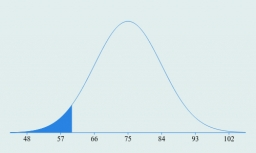Did you find an error or inaccuracy? Feel free to write us. Thank you!Tips to related online calculators
Looking for calculator of harmonic mean?
Looking for a statistical calculator?

## Related math problems and questions:

• Insert 7Insert five harmonic means between 3 and 18
• Insert 5Insert five harmonic means between 1/2 and 1/26
• Insert 6Insert four harmonic means between 3/7 and 3/19
• Harmonic mean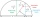If x, y, z form a harmonic progression, then y is the harmonic mean of x and z. Find the harmonic mean of the numbers 6 and 5.
• HP - harmonic progression 2Compute the 16th term of the HP if the 6th and 11th term of the harmonic progression are 10 and 18 respectively.
• Insert 3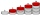Insert five arithmetic progression members between -7 and 3/2.
• Insert AP memberInsert arithmetic means between 75 and 180.
• Insert 4Insert three arithmetic means between 3 and 63.
• HP - harmonic progressionDetermine the 8th term of the harmonic progression 2, 4/3, 1,…
• Two geometric progressions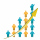Insert several numbers between numbers 6 and 384 so that they form with the given GP numbers and that the following applies: a) the sum of all numbers is 510 And for another GP to apply: b) the sum of entered numbers is -132 (These are two different geome
• HP - harmonic progressionDetermine the 10th term of the harmonic progression 6,4,3,…
• Right-angled triangle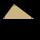Determine the content of a right triangle whose side lengths form successive members of an arithmetic progression and the radius of the circle described by the triangle is 5 cm.
• Insert into GPBetween numbers 5 and 640, insert as many numbers to form geometric progression so the sum of the numbers you entered will be 630. How many numbers must you insert?
• GP membersThe geometric sequence has 10 members. The last two members are 2 and -1. Which member is -1/16?
• Harmonic meanHarmonic means of 6 and 12
• Sum of GP members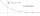Determine the sum of the GP 30, 6, 1.2, to 5 terms. What is the sum of all terms (to infinity)?
• Harmonic and arithmetic means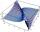The local Utah Department of Child Service office wants to project staffing needs based on current social worker assignments. They have the number of cases per social worker for the following staff: Mary: 25 John: 35 Ted: 15 Lisa: 45 Anna: 20 Calculate: a